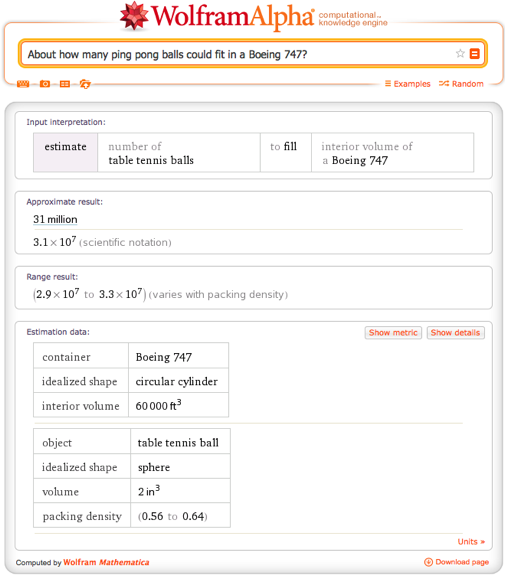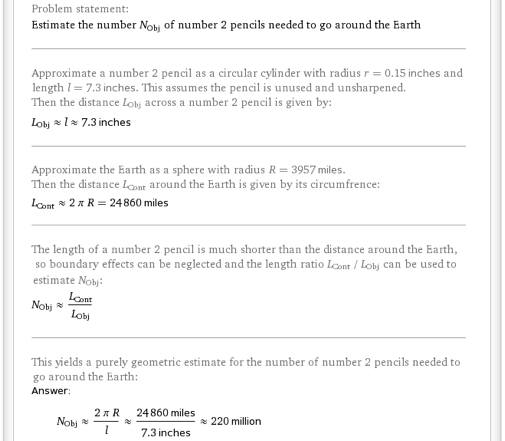# How Many Everyday Objects Would It Take To…

September 12, 2013 —Comments Off

As a physics major, I sometimes find myself solving interesting problems for fun. However, I have never been very quick at doing simple math in my head, so I often resort to using computers to do tedious calculations. This keeps me interested in the answer to the problem and not focused on the details of the calculations, which can be very boring. Computers are much faster at doing calculations than I am, and Wolfram|Alpha is no exception: for instance, arctan(3^4^3)/pi. However, many real-world problems cannot be solved with precision; sometimes a good estimation is the best that can be done.

This summer I was an intern for Wolfram|Alpha, adding a new set of functionality to the site that can solve order-of-magnitude estimation problems such as “About how many ping pong balls could fit in a Boeing 747?Wolfram|Alpha now knows simple geometric shapes, volumes, areas, and lengths of many common items, and it can use this information to answer estimation questions, including:

Not only does Wolfram|Alpha give a numerical answer, it also shows details about the calculation, including a Step-by-step solution to the problem by clicking the Show details button.We are working on adding more items for this feature, but if you want to do a calculation for an item of your own, you can enter the volume, area, or length of it instead:

The goal of estimation problems like these is to get an approximate answer, since all objects are not the same, and packing densities vary from combination to combination. To avoid such complications, we only consider simple geometry and ignore all other effects (including gravitational and thermal effects).

Here are some interesting questions to try out:

With this new functionality, you can use Wolfram|Alpha to get an idea of how many M&M’s might fit in a jar for a contest, to pack for your next road trip, or to just ask some interestingly wild questions for fun.

1 Comment

This is fantastic stuff, and the show steps feature is great. Nice work!

Posted by Daniel Bigham September 12, 2013 at 2:32 pm